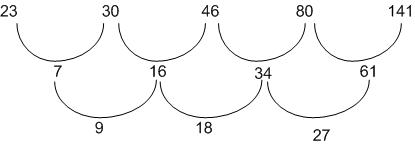# Number Series Questions for Bank Exams PDF

1
5758

Number series is the predominant topic for every banking exam. Number series questions usually appear in the reasoning section. Remembering some basic patterns will make this topic easy. But the questions will be mixture of easy, moderate and difficult questions. You should not spend most time on solving difficult number series questions. Within 15 to 20 seconds, you should judge whether you can solve it or not. This number series questions will be useful for all banking exams like IBPS Clerk, IBPS PO, SBI PO, SBI Clerk, IBPS SO, RBI.

# Number Series Questions for Bank Exams PDF:

Instructions:

What will come in place of the question mark (?) in the given number series

Question 1:

17, 19, 25, 37, ?, 87

a) 63
b) 52
c) 55
d) 67
e) 57

Question 2:

61, 82, 124, 187, ?, 376

a) 271
b) 263
c) 257
d) 287
e) 249

Question 3:

23, 30, 46, 80, 141, ?

a) 244
b) 212
c) 226
d) 220
e) 238

Question 4:

179, 180, 172, 199, 135, ?

a) 236
b) 272
c) 240
d) 256
e) 260

Question 5:

14, 6, 5, 6.5, 12, ?

a) 29
b) 27
c) 23
d) 33
e) 35

Question 6:

8 ? 16 48 192 960

a) 16
b) 10
c) 12
d) 14
e) 8

Question 7:

16.8 16.85 16.75 16.9 16.7 ?

a) 17.5
b) 17.25
c) 16.95
d) 16.25
e) 18.85

Question 8:

8 20 35 54 78 ?

a) 114
b) 169
c) 108
d) 123
e) 136

Question 9:

16 ? 32 16 4 0.5

a) 28
b) 20
c) 24
d) 32
e) 18

Question 10:

63 72 97 146 227 ?

a) 263
b) 466
c) 348
d) 329
e) 384

Number Series Questions for Bank Exams PDF with answers.

Solutions (1 to 10)

The pattern in the series is,
$17+1\times2=19$.
$19+2\times3=25$.
$25+3\times4=37$.
$37+4\times5=57$.
$57+5\times6=87$.
Hence, Option E is the correct answer.

The pattern in the series id,
$61 + 21 = 82$.
$82 + (21\times2) = 82 + 42 = 124$.
$124 + (21\times3) = 124 + 63 = 187$.
$187 + (21\times4) = 187 + 84 = 271$.
$271 + (21\times5) = 271 + 105 = 376$.
Hence, Option A is the correct answer.

The pattern followed by series is,Hence the next term will be = 61+36+141.
=238. Therefore, Option E is correct.

The series follows a pattern:
$179+1^{3}=180$.
$180-2^{3}=172$.
$172+3^{3}=199$.
$199-4^{3}=135$.
Hence, Next term in the series will be = $135+5^{3}=260$.
Therefore, Option E is correct.

14,6,5,6.5,12,?
Let unknown quantity be’x’.
The series is $(14\times0.5 – 1), (6 \times1 – 1), (5\times1.5 – 1), (6.5\times2 – 1),……$.
Hence,$x=12\times2.5-1$.
$x=29$.
Hence, Option A is correct.

8 $\times 1$ = 8
8 $\times 2$ = 16
16 $\times 3$ = 48
48 $\times 4$ = 192
192 $\times 5$ = 960

Multiples of 0.05 are alternatively added and subtracted

16.8 $+ 0.05 \times 1$ = 16.85
16.85 $- 0.05 \times 2$ = 16.75
16.75 $+ 0.05 \times 3$ = 16.9
16.9 $- 0.05 \times 4$ = 16.7
16.7 $+ 0.05 \times 5$ = 16.95

The pattern is :

8 + $12$ = 20
20 + $12 + 3$ = 35
35 + $12 + 3 + 4$ = 54
54 + $12 + 3 + 4 + 5$ = 78
78 + $12 + 3 + 4 + 5 + 6$ = 108

The pattern is :

16 $\times 2$ = 32
32 $\times 1$ = 32
32 $\times \frac{1}{2}$ = 16
16 $\times \frac{1}{4}$ = 4
4 $\times \frac{1}{8}$ = 0.5

Square of odd numbers are added

63 $+ 3^2$ = 72
72 $+ 5^2$ = 97
97 $+ 7^2$ = 146
146 $+ 9^2$ = 227
227 $+ 11^2$ = 348

We hope that this Number Series Questions for Bank Exams PDF with answers is useful to you.

#### 1 COMMENT

1.Randhir kumar